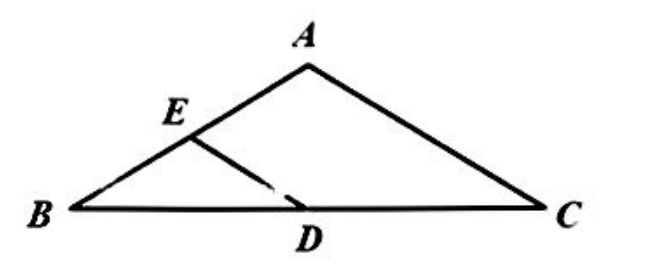$\text{A.}$ $\frac{1}{2}$ $\text{B.}$ $\frac{1}{4}$ $\text{C.}$ $\frac{3}{4}$ $\text{D.}$ $\frac{7}{8}$
【答案】 B